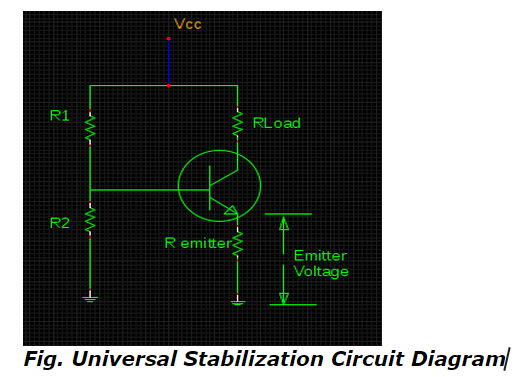# Universal Stabilization Circuit & V-I Notations - MCQs with answers

## Universal Stabilization Circuit & V-I Notations - MCQs with answers

Q1. What should be the combined value of resistance according to Beta rule especially when the value of βRE is greater than R2 in the following schematic configuration of Universal Stabilization Circuit?a. R2¦ βRE
b. R2¦ βRL
c. R1R2¦ βRE
d. R1R2¦ βRL

Q2. What does the notation of VBB ↓ , IB ↓ , IC ↓ , IC RL ↓ , VCE ↑ imply, according to the notation rules at incremental & decremental stages?

a. Decrease in input voltage increases the output voltage
b. Increase in input voltage decreases the output voltage
c. Increase in input voltage also increases the output voltage
d. Decrease in input voltage also decreases the output voltage

ANSWER: a. Decrease in input voltage increases the output voltage

Q3. Which is the standard form of representing the instantaneous values of a.c. currents in accordance to V-I notation rules?

a. IE, IB, IC
b. ie, ib, ic
c. Ie, Ib , Ic
d. All of the above